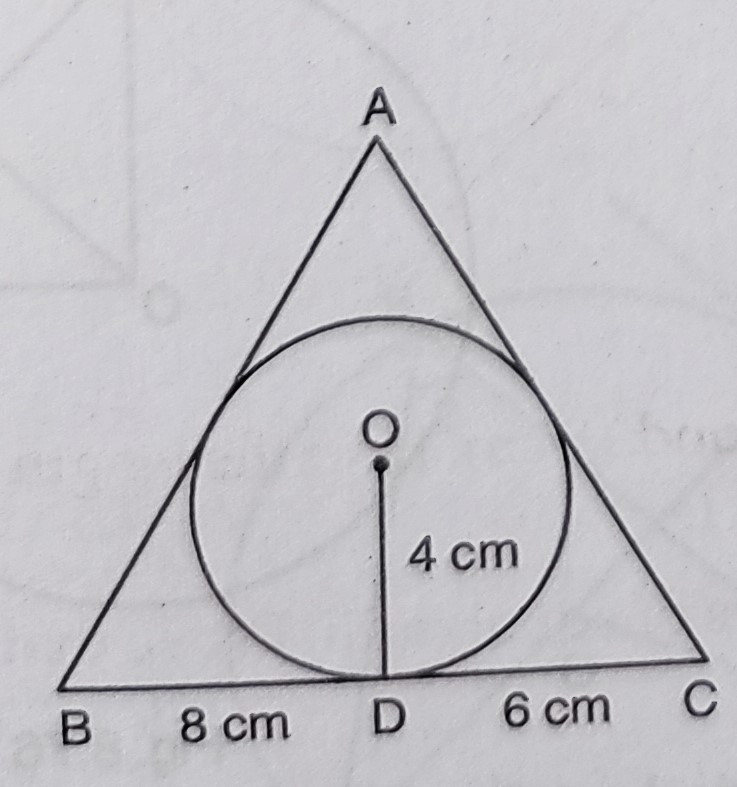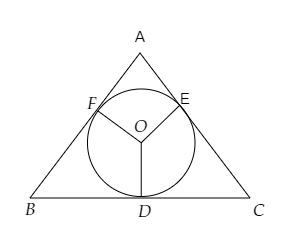# In the figure, a $\triangle A B C$ is drawn to circumscribe a circle of radius $4 \mathrm{~cm}$ such that the segments $B D$ and $D C$ are of lengths $8 \mathrm{~cm}$ and $6 \mathrm{~cm}$ respectively. Find the lengths of sides $A B$ and $A C$, when area of $\triangle A B C$ is $84 \mathrm{~cm}^{2}$."

Given:

In the figure, a $\triangle A B C$ is drawn to circumscribe a circle of radius $4 \mathrm{~cm}$ such that the segments $B D$ and $D C$ are of lengths $8 \mathrm{~cm}$ and $6 \mathrm{~cm}$ respectively.

Area of $\triangle A B C$ is $84 \mathrm{~cm}^{2}$.

To do: We have to find the lengths of sides $A B$ and $A C$.

Solution:In the given figure,

A circle is inscribed in $\triangle ABC$ touching it at $D, E$ and $F$ respectively.

Radius of the circle $r = 4\ cm$

$OD\ \perp\ BC$

$OD = 4\ cm, BD = 8\ cm, DC = 6\ cm$

Join $OE$ and $OF$.

Area of $\Delta \mathrm{ABC}=84 \mathrm{~cm}^{2}$

$=\frac{1}{2}$ (Perimeter of $\Delta \mathrm{ABC}) \times r$

$\Rightarrow \frac{1}{2} r \times$ (Perimeter) $=84$

Therefore,

Perimeter $=\frac{84 \times 2}{r}$

$=\frac{84 \times 2}{4}$

$=42 \mathrm{~cm}$

$\mathrm{BD}=\mathrm{BF}=8 \mathrm{~cm}$     (Tangents to the cirlce are equal)

Similarly,

$\mathrm{CD}=\mathrm{CE}=6 \mathrm{~cm}$

$A E=A F$

$B D+C E+A F=\frac{1}{2}$  (Perimeter)

$=\frac{1}{2} \times 42$

$=21 \mathrm{~cm}$

$\Rightarrow 8+6+A F=21$

$\Rightarrow A F=21-8-6=7$

$A E=A F=7 \mathrm{~cm}$

$A B=A F+F B$

$=7+8$

$=15 \mathrm{~cm}$

$A C=A E+E C$

$=7+6$

$=13 \mathrm{~cm}$

The lengths of sides $A B$ and $A C$ are $15\ cm$ and $13\ cm$ respectively.